Title# College Algebra

## Tutorial 39: Zeros of Polynomial Functions, Part II: Upper and Lower Bounds, Intermediate Value Theorem, Fundamental Theorem of Algebra, and the Linear Factorization TheoremLearning Objectives

 After completing this tutorial, you should be able to: Determine if a given number is an upper or lower bound for roots of a polynomial function. Use the Intermediate Value Theorem to approximate real zeros of polynomial functions. Know that if a non-real complex number is a root of a polynomial function that its conjugate is also a root. Know what the Fundamental Theorem of Algebra is. Use the Linear Factorization Theorem to find an nth degree polynomial function given its zeros.Introduction

 In this tutorial we will be looking at several aspects dealing with zeros of polynomial functions.  If you need a review on how to find zeros, the Rational Zero Theorem or Descartes' Rule of Signs, feel free to go to Tutorial 38: Zeros of Polynomial Functions, Part I.  On this page we dive a little deeper into the concept of zeros.  One thing that we will look at is finding the upper and lower bounds for roots of a polynomial function.  This can help us narrow down the possibilities of rational zeros.  Another concept on this page is the Intermediate Value Theorem.  This can help narrow down the possibilities of real zeros, especially those that land between integer values.  We will also work with non-real complex numbers.  Did you know that if a non-real complex number is a zero of a polynomial function, that its conjugate is too?   We will follow that up by using the Fundamental Theorem of Algebra and the Linear Factorization Theorem to find polynomial functions given zeros.  Wow, it looks like we have our work cut out for us.  I guess you better get started.Tutorial

 The Upper and Lower Bound Theorem

 Upper Bound If you divide a polynomial function f(x) by (x - c), where c > 0, using synthetic division and this yields all positive numbers, then c is an upper bound to the real roots of the equation f(x) = 0. Note that two things must occur for c to be an upper bound.  One is c > 0 or positive.  The other is that all the coefficients of the quotient as well as the remainder are positive.

 Lower Bound If you divide a polynomial function f(x) by (x - c), where c < 0, using synthetic division and this yields alternating signs, then c is a lower bound to the real roots of the equation f(x) = 0.  Special note that zeros can be either positive or negative.  Note that two things must occur for c to be a lower bound.  One is c < 0 or negative.  The other is that successive coefficients of the quotient and the remainder have alternating signs.Example 1: Show that all real roots of the equationlie between - 4 and 4.

 In other words, we need to show that - 4 is a lower bound and 4 is an upper bound for real roots of the given equation. Checking the Lower Bound: Lets apply synthetic division with - 4 and see if we get alternating signs:Note how c = -4 < 0 AND the successive signs in the bottom row of our synthetic division alternate.  You know what that means? - 4 is a lower bound for the real roots of this equation.

 Checking the Upper Bound: Lets apply synthetic division with 4 and see if we get all positive:Note how c = 4 > 0 AND the all of the signs in the bottom row of our synthetic division are positive.  You know what that means? 4 is an upper bound for the real roots of this equation.   Since - 4 is a lower bound and 4 is an upper bound for the real roots of the equation, then that means all real roots of the equation lie between - 4 and 4.

 The Intermediate Value Theorem If f(x) is a polynomial function and f(a) and f(b) have different signs, then there is at least one value, c, between a and b such that f(c) = 0.

 In other words, when you have a polynomial function and one input value causes the function to be positive and the other negative, then there has to be at least one value in between them that causes the polynomial function to be 0.  This works because 0 separates the positives from the negatives.  So to go from positive to negative or vice a versa you would have to hit a point in between that goes through 0.Example 2: Show that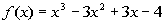has a real zero between 2 and 3.  Use the Intermediate Value theorem to find an approximation for this zero to the nearest tenth.

 When finding functional values, you can either use synthetic division or directly plug the number into the function.  Since we will only be interested in knowing the functional value in this problem, I’m going to directly plug my x value into the function.  If I were needing more, for example the signs of the quotient, like above, then I would use synthetic division.  Finding f(2):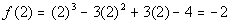Finding f(3):Since there is a sign change between f(2) = -2 and f(3) = 5, then according to the Intermediate Value Theorem, there is at least one value between 2 and 3 that is a zero of this polynomial function.   Checking functional values at intervals of one-tenth for a sign change:Finding f(2.1):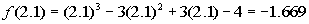Finding f(2.2):Finding f(2.3):Finding f(2.4):Finding f(2.5):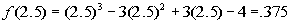Hey we have a sign change!!!!! Now we want to find the zero to the nearest tenth.  So is it going to be x = 2.4 or x = 2.5.  We can not necessarily go by which functional value is closer to zero.  We will need to dig a little bit deeper and go by intervals of one-hundredths:Finding f(2.41):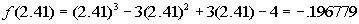Finding f(2.42):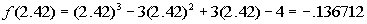Finding f(2.43):Finding f(2.44):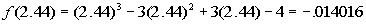Finding f(2.45):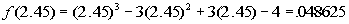Whew!!!!  At last we come to a sign change between successive hundredths.  That means we have it narrowed down at little bit better.  There is a zero between 2.44 and 2.45.  Since it would land slightly below 2.45, the nearest tenth would be 2.4. The work here is not hard, it is just tedious.

 Fundamental Theorem of Algebra Every polynomial function equation f(x) = 0 of degree one or higher has at least one complex root.

 Keep in mind that complex numbers include real numbers.  Real numbers are complex numbers where the imaginary part is 0.

 Conjugate Roots

 In polynomial function equations, non-real complex roots always occur in conjugate pairs. In other words, if a complex number with an imaginary part is a root of a polynomial function equation, then its conjugate is also a root of that same function.  Remember that a conjugate of a + bi is a - bi.  So, if 2 + 3i is a known root of a polynomial function equation, then 2 - 3i is also. If you need a review on complex numbers, feel free to go to Tutorial 12: Complex Numbers.

 The Linear Factorization Theorem If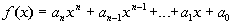where n > 1 and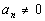thenwhereare complex numbers  (possibly real and not necessarily distinct)

 In other words, a polynomial function of degree n, where n > 0, can be factored into n (not necessarily distinct) linear factors over the complex number field.  Keep in mind that some factors may occur more than one time.  For each time a linear factor appears it is counted as a linear factor.  For example, if, the linear factor (x + 2) has a multiplicity of 3, which means that factor occurs three times.  So technically there are 4 linear factors, one (x - 3) and three (x + 2)’s.  This matches the degree of the polynomial function.Example 3: Use the given root to find all of the roots of the polynomial equation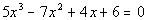;  1 + i.

 Since the complex number 1 + i is a root, that means it’s conjugate 1 - i is also a root.  This will help us break down the function to find any other roots. This is done in the same fashion as when you are given a real zero. If you need a review on finding roots of a polynomial equation f(x) = 0 when given a root, feel free to go to Tutorial 37: Synthetic Division and the Remainder and Factor Theorems. Using synthetic division to find the quotient we get:Yuck!!!   Look at all of those complex numbers in the quotient.  Don’t fear, when we put in our conjugate using that quotient those complex numbers will disappear and we will be left with a nice quotient with real number coefficients. Check it out:Now we are getting somewhere.  From here we can rewrite the original problem using the roots that we have above and the quotient that we ended up with in this last synthetic division.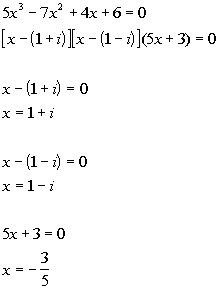*The 1st two factors are x minus the complex zeros *The 3rd factor is the quotient found directly above   *The given complex zero     *The conjugate zero *Setting the 3rd factor = 0

 So the roots of the polynomial equation are 1 + i, 1 - i and -3/5.Example 4:  Factor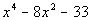a) as the product of factors that are irreducible over rational numbers, b) as the product of factors that are irreducible over real numbers, and c) in completely factored form involving complex non-real numbers.

 Factor as the product of factors that are irreducible over rational numbers: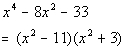*Factor the trinomial

 Since 11 is not a perfect square, this is as far as we can factor it using only rational numbers.   Factor as the product of factors that are irreducible over real numbers: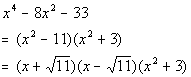*Factor the difference of squares

 Did you know that the sum of squares can be factored over the complex non-real numbers as? Completely factored form involving complex non-real numbers:Note how once we had factored over the complex numbers that we ended up with four linear factors and that our polynomial was degree four.

 Creating a Polynomial Function when Given Zeros

Now we are going to reverse things.  In the next two examples, we will be given zeros and the degree of a polynomial function, and we will need to find out what that polynomial is.

Step 1: Use the given zeros and the Linear Factorization Theorem to write out all of the factors of the polynomial function.

 Keep in mind that if you are given a non-real complex zero, that its conjugate is also a zero. Also keep in mind that the degree tells you how many linear factors over the complex numbers (possibly real and not necessarily distinct) that you will have.  The factors are written in the following way: if c is a zero than (x - c) is a factor of the polynomial function.

Step 2: Multiply all of the factors found in Step 1.Example 5:  Find an nth degree polynomial function where n = 3;  2 + 3i and 4 are zeros; f(3) = -20.

 Step 1: Use the given zeros and the Linear Factorization Theorem to write out all of the factors of the polynomial function.

 Since our degree is 3, that means there are three linear factors over the complex numbers (possibly real and not necessarily distinct). Note how we are only given two zeros.  We need to come up with a third one.  Do you have any ideas? Oh yeah, if a non-real complex number is a zero than its conjugate is also a zero.  Since 2 + 3i is a zero, that means 2 - 3i is also a zero. Using the Linear Factorization Theorem we get: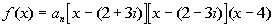Step 2: Multiply all of the factors found in Step 1.*Dist. - through comp. numbers *Multiply comp. factors *Simplify (i squared = -1) *Multiply remaining factors

 This problem gave another condition, f(3) = -20. This will help us find in this problem.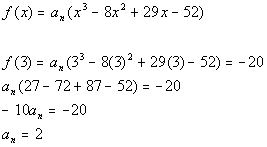*f(3) = -20 *Solve for a sub n

 Putting it all together we get: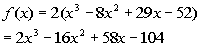Practice Problems

 These are practice problems to help bring you to the next level.  It will allow you to check and see if you have an understanding of these types of problems. Math works just like anything else, if you want to get good at it, then you need to practice it.  Even the best athletes and musicians had help along the way and lots of practice, practice, practice, to get good at their sport or instrument.  In fact there is no such thing as too much practice. To get the most out of these, you should work the problem out on your own and then check your answer by clicking on the link for the answer/discussion for that  problem.  At the link you will find the answer as well as any steps that went into finding that answer.Practice Problem 1a:  Show that all real roots of the given equation lie between -3 and 4.

 1a.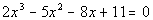(answer/discussion to 1a)Practice Problem 2a: Show that the given polynomial has a real zero between the given integers.  Use the Intermediate Value theorem to find an approximation for this zero to the nearest tenth.

 2a.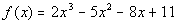;  between -1 and -2. (answer/discussion to 2a)Practice Problem 3a: Use the given root to find all of the roots of the given polynomial equation.

 3a.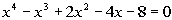;   2i (answer/discussion to 3a)Practice Problem 4a: Factor the given polynomial function  a) as the product of factors that are irreducible over rational numbers, b) as the product of factors that are irreducible over real numbers, and c) in completely factored form involving complex non-real numbers.

 4a.(answer/discussion to 4a)Practice Problem 5a: Find an nth degree polynomial function with the given conditions

 5a. n = 4; 2, -1, and 2i are zeros; f(1) = -30. (answer/discussion to 5a)Need Extra Help on these Topics?

 Go to Get Help Outside the Classroom found in Tutorial 1: How to Succeed in a Math Class for some more suggestions.

Last revised on March 15, 2012 by Kim Seward.